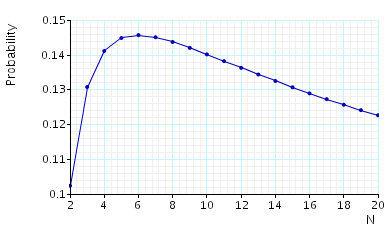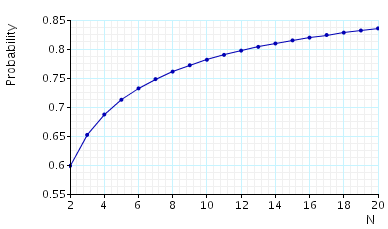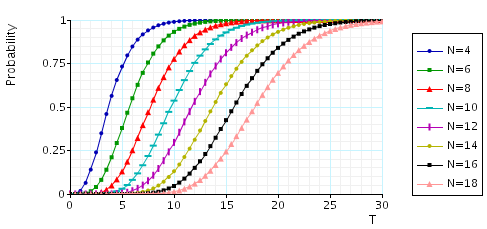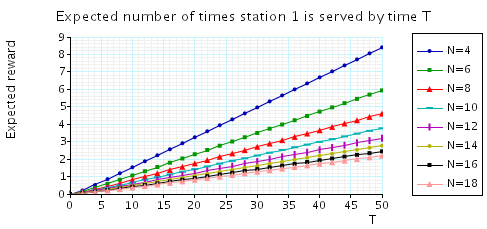# Cyclic Server Polling System

(Ibe and Trivedi)

### Introduction:

This case study is based on a cyclic server polling system, taken from [IT90]. The model is represented as a CTMC. We use N to denote the number of stations handled by the polling server. By way of example, the PRISM source code for the case where N=5 is given below.

```// polling example [IT90]
// gxn/dxp 26/01/00

ctmc

const int N=5;

const double mu= 1;
const double gamma= 200;
const double lambda= mu/N;

module server

s : [1..5]; // station
a : [0..1]; // action: 0=polling, 1=serving

[loop1a] (s=1)&(a=0) -> gamma	: (s'=s+1);
[loop1b] (s=1)&(a=0) -> gamma	: (a'=1);
[serve1] (s=1)&(a=1) -> mu	: (s'=s+1)&(a'=0);

[loop2a] (s=2)&(a=0) -> gamma	: (s'=s+1);
[loop2b] (s=2)&(a=0) -> gamma	: (a'=1);
[serve2] (s=2)&(a=1) -> mu	: (s'=s+1)&(a'=0);

[loop3a] (s=3)&(a=0) -> gamma	: (s'=s+1);
[loop3b] (s=3)&(a=0) -> gamma	: (a'=1);
[serve3] (s=3)&(a=1) -> mu	: (s'=s+1)&(a'=0);

[loop4a] (s=4)&(a=0) -> gamma	: (s'=s+1);
[loop4b] (s=4)&(a=0) -> gamma	: (a'=1);
[serve4] (s=4)&(a=1) -> mu	: (s'=s+1)&(a'=0);

[loop5a] (s=5)&(a=0) -> gamma	: (s'=1);
[loop5b] (s=5)&(a=0) -> gamma	: (a'=1);
[serve5] (s=5)&(a=1) -> mu	: (s'=1)&(a'=0);

endmodule

module station1

s1 : [0..1];

[loop1a] (s1=0) -> 1 : (s1'=0);
[]       (s1=0) -> lambda : (s1'=1);
[loop1b] (s1=1) -> 1 : (s1'=1);
[serve1] (s1=1) -> 1 : (s1'=0);

endmodule

// construct further stations through renaming
module station2 = station1 [s1=s2, loop1a=loop2a, loop1b=loop2b, serve1=serve2] endmodule
module station3 = station1 [s1=s3, loop1a=loop3a, loop1b=loop3b, serve1=serve3] endmodule
module station4 = station1 [s1=s4, loop1a=loop4a, loop1b=loop4b, serve1=serve4] endmodule
module station5 = station1 [s1=s5, loop1a=loop5a, loop1b=loop5b, serve1=serve5] endmodule

// cumulative rewards

rewards "waiting" // expected time the station 1 is waiting to be served
s1=1 & !(s=1 & a=1) : 1;
endrewards

rewards "served" // expected number of times station1 is served
[serve1] true : 1;
endrewards
```

### Model Statistics:

The table below shows statistics for each model we have built. The first section gives information about the CTMC representing the model: the number of states and the number of non-zeros (transitions). The second part refers to the MTBDD which represents this CTMC: the total number of nodes, the number of leaves (terminal nodes), and the amount of memory needed to store it. The last column gives the amount of memory a sparse matrix would need to represent the same CTMC.

 N: Model: MTBDD: Sparse: States: NNZ: Nodes: Leaves: Kb: Kb: 4 96 272 178 4 3.48 4.3 5 240 800 271 4 5.29 12.2 6 576 2,208 367 4 7.17 32.6 7 1,344 5,824 482 4 14.9 84 8 3,072 14,848 608 4 11.9 210 9 6,912 36,864 765 4 14.9 513 10 15,360 89,600 921 4 18.0 1,230 11 33,792 214,016 1,096 4 21.4 2,904 12 73,728 503,808 1,282 4 25.0 6,768 13 159,744 1,171,456 1,491 4 33.3 15,600 14 344,064 2,695,168 1,707 4 33.3 35,616 15 737,280 6,144,000 1,942 4 37.9 80,640 16 1,572,864 13,893,632 2,188 4 42.7 371,712 17 3,342,336 31,195,136 2,469 4 48.2 378,624 18 7,077,888 69,599,232 2,745 4 53.6 843,264 19 14,942,208 154,402,816 3,040 4 59.4 1,867,776 20 31,457,280 340,787,200 3,346 4 65.4 4,116,362

### Model Construction Times

The table below gives the times taken to construct the models. This process involves first building a CTMC (represented as an MTBDD) from the system description (in our module based language), and then computing the reachable states using a BDD based fixpoint algorithm. The total time required and the number of fixpoint iterations are given below.

 N: Construction: Time (s): Iter.s: 4 0.039 9 5 0.043 11 6 0.059 13 7 0.063 15 8 0.069 17 9 0.09 19 10 0.107 21 11 0.115 23 12 0.126 25 13 0.144 27 14 0.156 29 15 0.19 31 16 0.202 33 17 0.239 35 18 0.271 37 19 0.302 39 20 0.346 41

### Model Checking

We now report on the model checking results obtained using PRISM when checking the following CSL specifications.

```// Time bound
const double T;

// Probability that in the long run station 1 is awaiting service
S=? [ s1=1 & !(s=1 & a=1) ]

// Probability that in the long run station 1 is idle
S=? [ s1=0 ]

// Once a station becomes full, the minimum probability it will eventually be polled
P=? [ true U (s=1 & a=0) {s1=1}{min} ]

// Probability, from the inital state, that station 1 is served before station 2
P=? [ !(s=2 & a=1) U (s=1 & a=1) ]

// Probability that station 1 will be polled within T time units
P=?[ true U<=T (s=1 & a=0) ]

// Expected time that station 1 is waiting to be served
R{"waiting"}=?[C<=T]

// Expected number of times station1 is served
R{"served"}=?[C<=T] // number of times station1 is served
```

All experiments were carried out on a 2.80GHz PC with 1 Gb memory running Linux and in all iterative methods we stop iterating when the relative error between subsequent iteration vectors is less than 1e-6, that is:• Computing Steady State Probabilities: the table below gives the model checking statistics when computing these probabilities for both the Jacobi and Gauss-Seidel methods when using PRISM's different engines.

 N: Jacobi: Gauss Seidel: Iters: Time per iter: (s) Iters: Time per iter: (s) MTBDD Sparse Hybrid Sparse Hybrid 4 130 0.001100 < 0.00001 0.000015 18 < 0.00001 < 0.00001 5 173 0.002931 0.000012 0.000012 20 < 0.00001 < 0.00001 6 218 0.007445 0.000014 0.000028 22 < 0.00001 < 0.00001 7 264 0.020572 0.000042 0.000068 23 0.000087 0.000087 8 310 0.059178 0.000100 0.000158 25 0.000160 0.000320 9 358 0.256704 0.000257 0.000377 26 0.000462 0.000692 10 406 - 0.000643 0.000958 27 0.001037 0.001667 11 455 - 0.001545 0.002455 28 0.002393 0.003893 12 505 - 0.003612 0.006646 29 0.005207 0.009069 13 555 - 0.008295 0.015944 30 0.011733 0.020300 14 606 - 0.019363 0.035465 31 0.026161 0.045065 15 657 - 0.044633 0.080626 32 0.058656 0.104188 16 709 - 0.106413 0.191898 33 0.130212 0.231152 17 761 - 0.235858 0.409599 34 0.288147 0.537794 18 814 - 0.567683 0.908693 34 0.643882 1.187500 19 866 - 1.325487 2.003745 35 1.462714 2.633857 20 920 - - 4.664724 36 - 5.759333
• Probability that in the long run station 1 is awaiting service:• Probability that in the long run station 1 is idle:• Once station 1 becomes full, the minimum probability it will eventually be polled: the table below presents statistics for the model checking process. Note that as this property holds with probability 1 the statistics correspond to a BDD precomputation algorithm.

 N: Iters: Time per iter: (s) Minimum probability: 4 18 <0.000001 1 5 22 <0.000001 1 6 26 0.000385 1 7 30 0.000333 1 8 34 0.000294 1 9 38 0.000526 1 10 42 0.000714 1 11 46 0.000870 1 12 50 0.000800 1 13 54 0.001111 1 14 58 0.001207 1 15 62 0.001290 1 16 66 0.001515 1 17 70 0.001714 1 18 74 0.001757 1 19 78 0.002073 1 20 82 0.002308 1
• The probability that, from the initial state, station 1 is polled before station 2: the table and below gives the model checking statistics when computing these probabilities for both the Jacobi and Gauss-Seidel methods when using PRISM's different engines.

 N: Jacobi: Gauss Seidel: Iters: Time per iter: (s) Iters: Time per iter: (s) MTBDD Sparse Hybrid Sparse Hybrid 4 2,480 0.000463 0.000002 0.000002 1,862 0.000009 0.000011 5 2,664 0.000848 0.000005 0.000006 2,285 0.000021 0.000024 6 2,965 0.002041 0.000013 0.000016 2,479 0.000044 0.000045 7 3,124 0.005206 0.000030 0.000041 2,809 0.000095 0.000102 8 3,374 0.013286 0.000076 0.000101 2,965 0.000209 0.000233 9 3,516 0.033981 0.000180 0.000232 3,239 0.000455 0.000516 10 3,732 0.082460 0.000457 0.000560 3,374 0.000993 0.000956 11 3,863 0.193670 0.001076 0.001510 3,612 0.002220 0.002256 12 4,054 0.450005 0.002393 0.004357 3,734 0.004809 0.005239 13 4,176 1.047451 0.005484 0.009719 3,945 0.010381 0.009450 14 4,351 - 0.012999 0.022378 4,058 0.023012 0.021251 15 4,464 - 0.027970 0.049814 4,249 0.049110 0.047892 16 4,623 - 0.066075 0.111454 4,354 0.106876 0.106191 17 4,732 - 0.144911 0.247469 4,529 0.233834 0.243856 18 4,880 - 0.332425 0.554729 4,629 0.539982 0.535855 19 4,983 - 0.769429 1.226136 4,791 1.262000 1.185987 20 5,122 - - 2.666418 4,886 - 2.657864

The graph below we have plotted these probabilities as the number of stations varies.• The minimum probability that once station 1 is full it is polled within T time units: the table below presents statistics for the model checking process.

 N: T=5: T=50: Iters: Time per iter: (s) Iters: Time per iter: (s) MTBDD Sparse Hybrid MTBDD Sparse Hybrid 4 1,299 0.000108 0.000002 0.000004 3,029 0.000097 0.000002 0.000004 5 1,299 0.000360 0.000008 0.000012 3,390 0.000325 0.000006 0.000012 6 1,299 0.000833 0.000017 0.000033 3,735 0.000638 0.000016 0.000032 7 1,299 0.001525 0.000044 0.000089 4,068 0.001276 0.000040 0.000087 8 1,299 0.002905 0.000105 0.000226 4,393 0.002599 0.000100 0.000220 9 1,299 0.005907 0.000259 0.000557 4,709 0.005640 0.000245 0.000545 10 1,299 0.013120 0.000669 0.001400 5,019 0.012821 0.000612 0.001356 11 1,299 0.031202 0.001577 0.003540 5,324 0.030340 0.001483 0.003444 12 1,299 0.073051 0.003677 0.009074 5,625 0.069625 0.003399 0.008809 13 1,299 0.175253 0.008447 0.021134 5,921 0.151677 0.007790 0.020601 14 1,299 0.447443 0.019534 0.048891 6,213 0.350896 0.018084 0.049279 15 1,299 1.332977 0.045169 0.111782 6,503 0.882448 0.041978 0.109156 16 1,299 4.823504 0.109159 0.255657 6,789 2.453282 0.101669 0.249149 17 1,299 - 0.236249 0.574657 7,073 - 0.220543 0.559372 18 1,299 - 0.572048 1.288513 7,355 - 0.538752 1.253214 19 1,299 - 1.376863 2.853521 7,634 - 1.248819 2.778883 20 1,299 - - 6.311027 7,911 - - 6.146424

In the graph below, we plot results of the above property for different numbers of stations (values of N):• Expected time station 1 spends awaiting service by time T: the table and graph below we present the model checking statistics as both the number of stations and time bound T varies.

 N: T=5: T=50: Iters: Time per iter: (s) Iters: Time per iter: (s) MTBDD Sparse Hybrid MTBDD Sparse Hybrid 4 1,299 0.000270 0.000003 0.000006 3,856 0.000266 0.000004 0.000006 5 1,299 0.000653 0.000008 0.000017 4,766 0.000656 0.000009 0.000017 6 1,299 0.001532 0.000022 0.000040 5,677 0.001534 0.000025 0.000044 7 1,299 0.003857 0.000059 0.000107 6,585 0.003828 0.000063 0.000112 8 1,299 0.010498 0.000142 0.000266 7,489 0.010605 0.000150 0.000276 9 1,299 0.027769 0.000342 0.000649 8,391 0.028500 0.000361 0.000676 10 1,299 0.070794 0.000841 0.001601 9,290 0.075488 0.000865 0.001653 11 1,299 0.175307 0.001948 0.004038 10,187 0.207029 0.002015 0.004120 12 1,299 0.473611 0.004470 0.010046 11,082 0.951248 0.009620 0.010143 13 1,299 1.264613 0.010242 0.023289 11,111 3.642436 0.010285 0.023461 14 1,299 - 0.023290 0.053094 11,111 - 0.023422 0.053808 15 1,299 - 0.052793 0.120839 11,112 - 0.053346 0.121939 16 1,299 - 0.124015 0.273636 11,112 - 0.126152 0.276256 17 1,299 - 0.269986 0.611949 11,112 - 0.274005 0.616791 18 1,299 - 0.639747 1.400105 11,112 - 0.650463 1.371019 19 1,299 - - 3.016768 11,112 - - 3.030094 20 1,299 - - 6.629515 11,112 - - 6.676664• Expected number of times station 1 is served by time T: